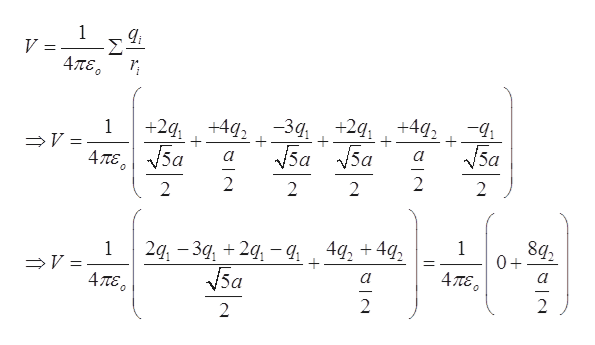# +442+24a+21+442

Question
223 views

The figure shows a rectangular array of charged particles fixed in place, with distance a = 33.7 cm and the charges shown as integer multiples of q1 = 3.32 pC and q2 = 7.25 pC. With V= 0 at infinity, what is the net electric potential at the rectangle's center?

check_circle

Step 1

The distance between each charge, a = 33.7 cm = 0.337 m

Charges shown in the array are multiples of, q1=3.32 pC = 3.32 x 10-12C and , q2=7.25 pC= 7.25 x 10-12C

We need to find the electric potential at the center of the rectangular array.

Step 2

The electric potential due to charges arranged in the rectangular array can be calculated by finding the potential due to individual charges at the center and then adding them up.help_outlineImage Transcriptionclose1 4TE -3q,+2 a 5a +442 + +2q,+4 a 1 + 5a + V 4 тE, 2 2 2 2 2 2 1 0+ 4q2 4q2 2q, - З4, + 29, —4 1 4TE Va 47TE 2 2 2 fullscreen
Step 3

Substitute the ...

### Want to see the full answer?

See Solution

#### Want to see this answer and more?

Solutions are written by subject experts who are available 24/7. Questions are typically answered within 1 hour.*

See Solution
*Response times may vary by subject and question.
Tagged in

### Electric Charges and Fields